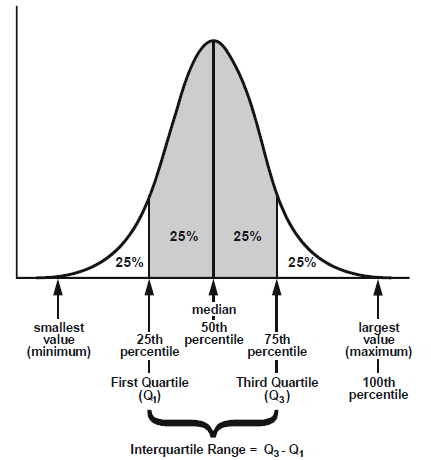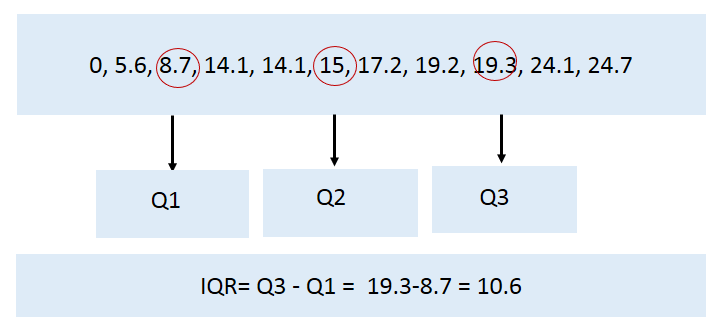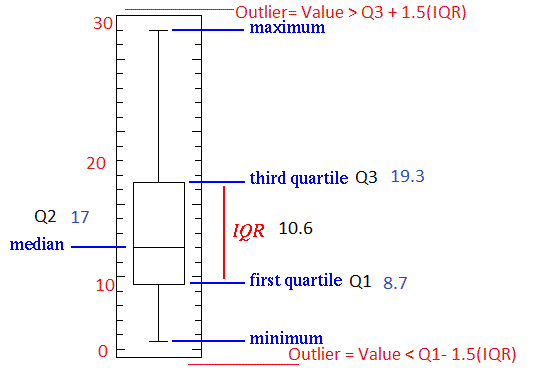### Range, Interquartile Range and Box Plot

Let’s think, in certain cases, you are comparing two groups. You have already calculated the central tendency of your data i.e. Mean, Median and Mode for both the groups. Sometimes it may happen that mean, median, and mode are same for both groups. Let’s take the below example:If you consider both the team their Mode= 14.1, Median=15 and Mean=15

This indicates that, if you adequately describe a distribution some time it may need more information than the measures of central tendency.

In this situation measures of variability comes into picture. They are

• Range
• Interquartile range.
• Box Plot to get good indication of how the values in a distribution are spread out.

#### Range:

The most simple measure of variability is the range. It is the difference between the highest and the lowest value.

For the above Example range will be:

Range(team1) = 19.3 – 10.8 = 8.5

Range(team2) = 27.7-0  = 27.7

As ranges takes only the count of extreme values sometimes it may not give you a good impact on variability. In this case, you can go for another measure of variability called interquartile range (IQR).

#### Interquartile Range (IQR):

Interquartile range gives another measure of variability. It is a better measure of dispersion than range because it leaves out the extreme values. It equally divides the distribution into four equal parts called quartiles. First 25% is 1st quartile (Q1), last one is 3rd quartile (Q3) and middle one is 2nd quartile (Q2).

2nd quartile (Q2) divides the distribution into two equal parts of 50%. So, basically it is same as Median.The interquartile range is the distance between the third and the first quartile, or, in other words, IQR equals Q3 minus Q1

IQR = Q3- Q1

##### How to calculate IQR

Step 1: Order from low to high

Step 2: Find the median or in other words Q2

Step 3: Then find Q1 by looking the median of the left side of Q2

Steps 4: Similarly find Q3 by looking the median of the right of Q2

Steps 5: Now subtract Q1 from Q3 to get IQR.

##### Example:

Consider the below example to get clear idea.Consider another example to get better understanding.

Consider the following numbers: 1, 3, 4, 5, 5, 6, 7, 11. Q1 is the middle value in the first half of the data set. Since there are an even number of data points in the first half of the data set, the middle value is the average of the two middle values; that is, Q1 = (3 + 4)/2 or Q1 = 3.5. Q3 is the middle value in the second half of the data set. Again, since the second half of the data set has an even number of observations, the middle value is the average of the two middle values; that is, Q3 = (6 + 7)/2 or Q3 = 6.5. The interquartile range is Q3 minus Q1, so IQR = 6.5 – 3.5 = 3.

##### Advantage of IQR:
• The main advantage of the IQR is that it is not affected by outliers because it doesn’t take into account observations below Q1 or above Q3.
• It might still be useful to look for possible outliers in your study.
• As a rule of thumb, observations can be qualified as outliers when they lie more than 1.5 IQR below the first quartile or 1.5 IQR above the third quartile.

Outliers = Q1 – 1.5* IQR   OR

=Q3 + 1.5*IQR

#### Box Plot:

There is one graph that is mainly used when you are describing center and variability of your data.

It is also useful for detecting outliers in the data.

Carefully, observe the above first IQR example when it is plotted in a boxplot.